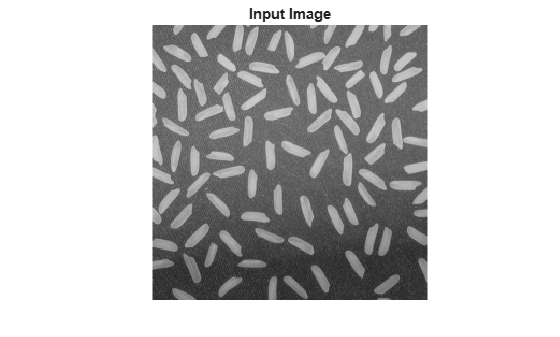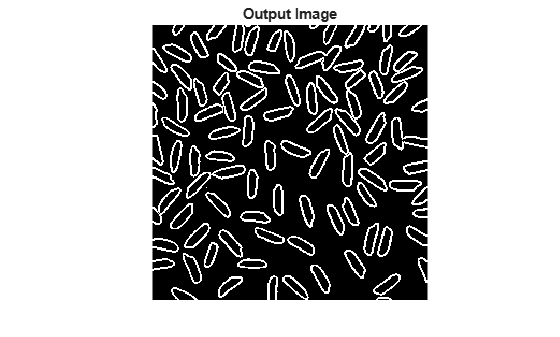# visionhdl.EdgeDetector

Find edges of objects

## Description

The `visionhdl.EdgeDetector` System object™ finds the edges in a grayscale pixel stream using the Sobel, Prewitt, or Roberts method. The object convolves the input pixels with derivative approximation matrices to find the gradient of pixel magnitude along two orthogonal directions. It then compares the sum of the squares of the gradients to a configurable threshold to determine if the gradients represent an edge. The Sobel and Prewitt methods calculate the gradient in horizontal and vertical directions. The Roberts method calculates the gradients at 45 and 135 degrees.

The object returns a binary image as a stream of pixel values. A pixel value of 1 indicates that the pixel is an edge. You can optionally enable output of the gradient values in the two orthogonal directions at each pixel.

To find the edges of objects:

1. Create the `visionhdl.EdgeDetector` object and set its properties.

2. Call the object with arguments, as if it were a function.

## Creation

### Syntax

``detectEdges = visionhdl.EdgeDetector``
``detectEdges = visionhdl.EdgeDetector(Name,Value)``

### Description

example

````detectEdges = visionhdl.EdgeDetector` returns a System object that detects edges using the Sobel method.```
````detectEdges = visionhdl.EdgeDetector(Name,Value)` returns an edge detector System object with properties specified using one or more name-value pairs. Enclose each property name in single quotes.```

## Properties

expand all

Unless otherwise indicated, properties are nontunable, which means you cannot change their values after calling the object. Objects lock when you call them, and the `release` function unlocks them.

If a property is tunable, you can change its value at any time.

When you select `'Sobel'` or `'Prewitt'`, the object calculates horizontal and vertical gradients, `Gv` and `Gh`. When you select `'Roberts'`, the object calculates orthogonal gradients, `G45` and `G135`. For details of each method, see Algorithms.

Note

When you select `'Prewitt'`, the full-precision internal data type is large due to the 1/6 coefficient. Consider setting the `GradientComponentOutputPorts` property to `true` so that you can customize the data type to a smaller size.

When this property is `true`, the object returns a binary pixel value representing whether an edge was detected at each location in the frame.

You must set at least one of `BinaryImageOutputPort` or `GradientComponentOutputPorts` to `true`. These properties specify the output arguments that return the edge results. If both properties are `false`, the object cannot return the results.

When this property is `true`, the object returns the `G1` and `G2` arguments representing the gradients calculated in two orthogonal directions at each pixel. When you set the `Method` property to `'Sobel'` or `'Prewitt'`, the outputs are horizontal and vertical gradients, `Gv` and `Gh`. When you set the `Method` property to `'Roberts'`, the outputs are diagonal orthogonal gradients, `G45` and `G135`. Set the data type for these arguments in the `GradientDataType` property.

You must set at least one of `BinaryImageOutputPort` and `GradientComponentOutputPorts` to `true`. These properties specify the output arguments that return the edge results. If both properties are `false`, the object cannot return the results.

Set this property to `'Input port'` to set the threshold as an input argument to the object. When this property is set to `'Property'`, set the threshold in the `Threshold` property.

The object compares the square of this value to the sum of the squares of the gradients. The object casts this value to the data type of the gradients. This property applies when you set `ThresholdSource` to `'Property'`.

The software supports `double` and `single` data types for simulation, but not for HDL code generation.

Data Types: `single` | `double` | `int` | `uint` | `fi`

Size of the line memory buffer, specified as a positive integer. Choose a power of two that accommodates the number of active pixels in a horizontal line. If you specify a value that is not a power of two, the buffer uses the next largest power of two.

The object allocates (N – 1)-by-`LineBufferSize` memory locations to store the pixels, where N is the number of lines in the differential approximation matrix. If you set the `Method` property to `'Sobel'` or `'Prewitt'`, then N is 3. If you set the `Method` property to `'Roberts'`, then N is 2.

• `'Symmetric'` — Set the value of the padding pixels to mirror the edge of the image. This option prevents edges from being detected at the boundaries of the active frame.

• `'None'` — Exclude padding logic. The object does not set the pixels outside the image frame to any particular value. This option reduces the hardware resources that are used by the object and reduces the blanking that is required between frames. However, this option affects the accuracy of the output pixels at the edges of the frame. To maintain pixel stream timing, the output frame is the same size as the input frame. To avoid using pixels calculated from undefined padding values, mask off the n/2 pixels around the edge of the frame for downstream operations. n is the size of the operation kernel. For more details, see Increase Throughput by Omiting Padding.

When the input is any integer or fixed-point data type, the algorithm uses fixed-point arithmetic for internal calculations. This property does not apply when the input data type is `single` or `double`.

The object uses fixed-point arithmetic for internal calculations when the input is any integer or fixed-point data type. This option does not apply when the input data type is `single` or `double`.

• `'Full precision'` (default) — Use full-precision rules based on the data type of the `pixelIn` argument, and the coefficients of the derivative approximation matrices.

• `'custom'` — Use the data type defined in the `CustomGradientDataType` property.

Data type for the gradient output values, specified as `numerictype(signed,WL,FL)`, where WL is the word length and FL is the fraction length in bits.

## Usage

### Syntax

``[edge,ctrlout] = detectEdges(pixelin,ctrlin)``
``[G1,G2,ctrlout] = detectEdges(pixelin,ctrlin)``
``[edge,ctrlout] = detectEdges(pixelin,ctrlin,thresh)``

### Description

example

````[edge,ctrlout] = detectEdges(pixelin,ctrlin)` detects edges in the neighborhood of `pixelin` by computing the gradient in two orthogonal directions. The `edge` output argument is a binary value indicating whether the sum of the squares of the gradients for the input pixel is above the threshold indicating an edge.```
````[G1,G2,ctrlout] = detectEdges(pixelin,ctrlin)` detects edges in the neighborhood of `pixelIn` by computing the gradient in two orthogonal directions. Use this syntax when you set the `GradientComponentOutputPorts` property to `true`. The `G1` and `G2` output arguments are the gradients calculated in the two orthogonal directions. When you set the `Method` property to '`Sobel'` or `'Prewitt'`, the first argument is the vertical gradient, and the second argument is the horizontal gradient. When you set the `Method` property to `'Roberts'`, the first argument is the 45-degree gradient, and the second argument is the 135-degree gradient.```
````[edge,ctrlout] = detectEdges(pixelin,ctrlin,thresh)` detects edges in the neighborhood of `pixelin` by computing the gradient in two orthogonal directions. Use this syntax when you set the `ThresholdSource` property to `'InputPort'`. The `edge` output argument is a binary value indicating whether the sum of the squares of the gradients is above the threshold, `thresh`, squared.```

You can use any combination of the optional argument syntaxes.

This object uses a streaming pixel interface with a structure for frame control signals. This interface enables the object to operate independently of image size and format and to connect with other Vision HDL Toolbox™ objects. The object accepts and returns a scalar pixel value and control signals as a structure containing five signals. The control signals indicate the validity of each pixel and its location in the frame. To convert a pixel matrix into a pixel stream and control signals, use the `visionhdl.FrameToPixels` object. For a description of the interface, see Streaming Pixel Interface.

### Input Arguments

expand all

Intensity of a single pixel, specified as a scalar value.

The software supports `double` and `single` data types for simulation, but not for HDL code generation.

You can simulate System objects with a multipixel streaming interface, but you cannot generate HDL code for System objects that use multipixel streams. To generate HDL code for multipixel algorithms, use the equivalent Simulink® blocks.

Data Types: `int` | `uint` | `fi` | `single` | `double`

Control signals accompanying the input pixel stream, specified as a `pixelcontrol` structure containing five `logical` data type signals. The signals describe the validity of the pixel and its location in the frame. For more details, see Pixel Control Structure.

Data Types: `struct`

Gradient threshold value that indicates an edge, specified as a scalar numeric value.

The object compares this value squared to the sum of the squares of the gradients.

#### Dependencies

To enable this argument, set `ThresholdSource` property to `'InputPort'`.

Data Types: `int` | `uint` | `fi` | `single` | `double`

### Output Arguments

expand all

Pixel value indicating an edge at this pixel, returned as a scalar binary value.

Gradient calculated in the first direction, returned as a scalar value.

If you set the `Method` property to `'Sobel'` or `'Prewitt'`, this argument is the vertical gradient. When you set the `Method` property to `'Roberts'`, this argument is the 45-degree gradient.

Configure the data type of the gradients by using the `GradientComponentDataType` and `CustomGradientComponent` properties.

#### Dependencies

To enable this argument, set the `GradientComponentOutputPorts` property to `true`

Gradient calculated in the second direction, returned as a scalar value.

If you set the `Method` property to `'Sobel'` or `'Prewitt'`, this argument is the horizontal gradient. When you set the `Method` property to `'Roberts'`, this argument is the 135-degree gradient.

Configure the data type of the gradients by using the `GradientComponentDataType` and `CustomGradientComponent` properties.

#### Dependencies

To enable this argument, set the `GradientComponentOutputPorts` property to `true`.

Control signals accompanying the output pixel stream, returned as a `pixelcontrol` structure containing five `logical` data type signals. The signals describe the validity of the pixel and its location in the frame. For more details, see Pixel Control Structure.

Data Types: `struct`

## Object Functions

To use an object function, specify the System object as the first input argument. For example, to release system resources of a System object named `obj`, use this syntax:

`release(obj)`

expand all

 `step` Run System object algorithm `release` Release resources and allow changes to System object property values and input characteristics `reset` Reset internal states of System object

## Examples

collapse all

Detect edges in a thumbnail image by using the Sobel method.

Import a test image.

```frmInput = imread('rice.png'); [frmActivePixels,frmActiveLines] = size(frmInput); figure imshow(frmInput) title 'Input Image'```Create a serializer and specify the size of inactive pixel regions.

```frm2pix = visionhdl.FrameToPixels( ... 'NumComponents',1, ... 'VideoFormat','custom', ... 'ActivePixelsPerLine',frmActivePixels, ... 'ActiveVideoLines',frmActiveLines, ... 'TotalPixelsPerLine',frmActivePixels+10, ... 'TotalVideoLines',frmActiveLines+10, ... 'StartingActiveLine',6, ... 'FrontPorch',5);```

Create an edge detection object with the default property values. The default detection method is Sobel.

`edgeDetectSobel = visionhdl.EdgeDetector();`

Speed up simulation by enabling a simulation mode that uses code generation for the System object.

`edgeDetectSobel.simulateUsing('Code generation');`

Serialize the test image by using the object you created. `pixIn` is a vector of intensity values. `ctrlIn` is a vector of control signal structures. Preallocate vectors for the output signals.

```[pixIn,ctrlIn] = frm2pix(frmInput); [~,~,numPixelsPerFrame] = getparamfromfrm2pix(frm2pix); ctrlOut = repmat(pixelcontrolstruct,numPixelsPerFrame,1); edgeOut = false(numPixelsPerFrame,1);```

For each pixel in the stream, compute whether it represents an edge.

```for p = 1:numPixelsPerFrame [edgeOut(p),ctrlOut(p)] = edgeDetectSobel(pixIn(p),ctrlIn(p)); end```

Create a deserializer with a format matching that of the serializer. Use the deserializer to convert the output pixel stream to an image frame.

```pix2frm = visionhdl.PixelsToFrame( ... 'NumComponents',1, ... 'VideoFormat','custom', ... 'ActivePixelsPerLine',frmActivePixels, ... 'ActiveVideoLines',frmActiveLines, ... 'TotalPixelsPerLine',frmActivePixels+10); [frmOutput,frmValid] = pix2frm(edgeOut,ctrlOut); if frmValid figure imshow(frmOutput) title 'Output Image' end```## Algorithms

This object implements the algorithms described on the Edge Detector block reference page.

## Version History

Introduced in R2015a

expand all